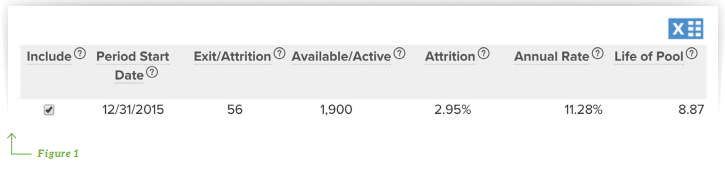The FASB’s Current Expected Credit Loss (CECL) model presents unique challenges for banking professionals. To help institutions prepare, Abrigo launched a CECL webinar series covering data, segmentation, methodology, and forecasting requirements broken down by loan pool-type. A key component of the series is allowing participants to ask their CECL-related questions, and below are several questions and answers relating to contractual life.

Questions related to contractual life under CECL:How do you calculate the average life for the loan? How it is related to attrition analysis?
Taking Figure 1 as an example, this mortgage pool with 1900 loans has had a quarterly attrition # of 56 loans. To get the attrition rate of 2.95%, you would take 56 and divide it by the total loans in the pool, 1900, which when annualized gives you 11.28% percentage. This is an effective interest calculation of that quarterly rate. It is okay to assume that this attrition rate is linear for your forecasting purposes, which when carried through gives you an average life of 8.87 years.

Is attrition count based or dollar based?
You could argue this either way. At the end of the day we are trying to answer, what is the probability of these loans exiting, how many are exiting and at what pace?

In a sample of N equally likely outcomes, mathematicians assign a chance (or weight) of 1/N to each outcome.

The probability of an event is defined as the number of certain outcomes divided by the total number of equally likely outcomes in the sample space of the experiment.

Using a dollar based approach implies an already determined (supportable) correlation to attrition rates.
– 11 Loans; 10 @ \$1, and 1 @ \$90. \$90 exits the portfolio over the analysis period. Should we then assume 9 of the remaining 10 loans will exit in the following quarter?

– If dollars are correlated to behavior, that implies a risk variance between loans within a pool that is, by definition, intended to contain loans with similar risk characteristics.

When do we use a prepayment rate such as CPR and SMM and when do we use expected life?
You would use a prepayment rate if you were using a discounted cashflow (DCF) approach. A prepayment would not be important when you use a Vintage or Migration approach, and likewise an attrition number is less important for DCF.

How do you calculate the prepayment rate? Can you go into more detail on how it is calculated?
A lot of banks do this internally, and others contract it out as a part of their ALM process. A typical way to perform this is to look at your loan performance over a certain set of historical months and compare loan performance to expected loss.

If you are interested in more answers to CECL, watch the on-demand webinar, CECL Methodology Q&A.### Sageworks

Raleigh, N.C.-based Sageworks, a leading provider of lending, credit risk, and portfolio risk software that enables banks and credit unions to efficiently grow and improve the borrower experience, was founded in 1998. Using its platform, Sageworks analyzed over 11.5 million loans, aggregated the corresponding loan data, and created the largest